# Numpy Factorial, Explained

In this tutorial, I’ll explain how to use the Numpy factorial function, AKA np.math.factorial.

I’ll explain the syntax of np.math.factorial, how the function works, and how to use it.

I’ll also show you how to use a different function, scipy.special.factorial, to compute factorials element-wise on Numpy arrays.

Having said that, it’s probably best if you read the whole tutorial. Everything will probably make more sense that way.

## A Quick Introduction to Numpy Factorial

First of all, let’s just start off with an overview of factorials, and how we can compute factorials with Numpy and Scipy.

### A Quick Review of Factorials

Quickly, let’s review what factorials are.

The factorial of an integer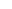is denoted as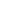, and is defined as:

(1)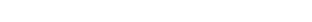So as an example we can compute the factorial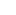as:

(2)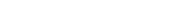Now, let’s talk about how to compute factorials in Python and Numpy.

### There are several important ways to compute factorials in Python

For better or worse, there’s not one single way to compute factorials in Python or in Numpy.

If you want to compute a Numpy factorial value, I recommend two functions:

• numpy.math.factorial()
• scipy.special.factorial()

The numpy.math.factorial() function only works for single integer values.

If you want to compute factorials on an array of values, you need to use scipy.special.factorial().

Let’s take a look at the syntax for both, and then I’ll show you some examples.

## Numpy.math.factorial syntax

The syntax for the Numpy factorial function is simple:Remember: this assumes that you’ve imported Numpy with the code import numpy as np.

It’s important to note that the Numpy factorial function (AKA, numpy.math.factorial) only accepts integer values as an input. It will throw an error if you attempt to use this function on a Numpy array.

If you want to compute factorials on all of the values of a Numpy array, you need to use a different function.

## scipy.special.factorial syntax

If you want to compute factorials on an entire Numpy array, you need to use scipy.special.factorial() function.

To use this function, you need to import scipy.special with the code import scipy.special.

Once you do that, you can use scipy.special.factorial(). The syntax is as follows:Notice that when you’re using scipy.special.factorial(), the input is more flexible. In particular, scipy.special.factorial() will accept inputs in the form of:

• integers
• Numpy arrays filled with integers

That being the case, scipy.special.factorial() is actually more flexible.

## Examples: how to calculate factorials in Numpy and Scipy

Now, let’s take a look at some examples.

Examples:

### EXAMPLE 1: Use the Numpy factorial function on an integer

Here, we’re going to use the Numpy factorial function (i.e., numpy.math.factorial).

#### Import Numpy

First, if you haven’t done so already, you need to import Numpy with the following code:

import numpy as np


#### Calculate Factorial with Numpy

Now, we can use Numpy to calculate the factorial of an integer.

Here, we’ll calculate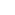.

np.math.factorial(5)


OUT:

120


#### Explanation

This is really straight forward.

Here, we’re providing the integer 5 as the input to the function.

The function computes the factorial: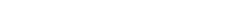.

One important thing to reiterate here is that np.math.factorial() will not work on floating point numbers or Numpy arrays.

To calculate the factorials on a Numpy array, you need to use a different function.

### EXAMPLE 2: Calculate the Factorials for the Values of a Numpy array

Now, we’re going to use scipy.special.factorial() to calculate the factorials of values of a Numpy array.

To do this, we’ll need to import scipy.special, and also create a Numpy array.

#### Import packages

First, we need to import numpy and scipy.special.

You can do that with the following code:

import numpy as np
import scipy.special


#### Create Numpy Array

Next, we’ll use the Numpy array function to create a simple numpy array with 6 values:

my_array = np.array([[1,2,3],[4,5,6]])


Let’s quickly take a look at the array, so you can see the contents:

print(my_array)


OUT:

[[1 2 3]
[4 5 6]]


As you can see, this Numpy array which I’ve called my_array contains the integer values from 1 to 6, arranged in an array with two rows and three columns.

#### Calculate Factorial of the Numpy array values

Now, we’ll use scipy.special.factorial() to calculate the factorials of each integer in our Numpy array:

scipy.special.factorial(my_array)


OUT:

array([[  1.,   2.,   6.],
[ 24., 120., 720.]])


#### Explanation

This is pretty simple.

Here, we’ve provided my_array as the input to scipy.special.factorial().

In the output, scipy.special.factorial() has calculated the factorial values of each value in the input array.So it’s pretty simple. You can try this on larger arrays as well, and for larger integers, but be aware that it may have large computation costs.

Do you have other questions about how to compute factorials in Numpy or Scipy?

In this tutorial, I’ve explained how to compute factorials in Numpy and Scipy.

This should help you with factorials in Numpy, but if you really want to learn Numpy, there’s a lot more to learn.

If you’re serious about mastering Numpy, and serious about data science in Python, you should consider joining our premium course called Numpy Mastery.

Numpy Mastery will teach you everything you need to know about Numpy, including:

• How to create Numpy arrays
• How to reshape, split, and combine your Numpy arrays
• What the “Numpy random seed” function does
• How to use the Numpy random functions
• How to perform mathematical operations on Numpy arrays
• and more …

Moreover, this course will show you a practice system that will help you master the syntax within a few weeks. We’ll show you a practice system that will enable you to memorize all of the Numpy syntax you learn. If you have trouble remembering Numpy syntax, this is the course you’ve been looking for.

Find out more here:

Joshua Ebner

Joshua Ebner is the founder, CEO, and Chief Data Scientist of Sharp Sight. Prior to founding the company, Josh worked as a Data Scientist at Apple. He has a degree in Physics from Cornell University.

### 1 thought on “Numpy Factorial, Explained”

1. You might want to explain that for numbers larger than so and so, you can use Stirling’s approximation. That is a requirement since factorial with integers can only go so far.